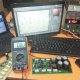Breaking News
Home / making transformer / Step-down Transformer

# Step-down Transformer

## How to make Step-down Transformer:

At first, we need to know how to make transformer? To make a transformer we need to know where we are going to use it. how many Voltage, Watts and ampere we need. Before all, we must know about the wire gauge for ampere.Gauge     –      Ampere

7              –        44.2

8              –        33.3

9              –        26.5

10            –        21.2

11            –        16.6

12            –        13.5

13            –        10.5

14            –          8.3

15            –          6.6

16            –          5.2

17            –          4.1

18            –          3.2

19            –          2.6

20            –          2.0

21            –          1.6

22            –          1.2

23            –          1.0

24            –          0.8

25            –          0.6

26            –          0.5

27            –          0.4

28            –          0.3

29            –          0.29

30            –          0.22

First, we have to know about voltage, ampere, and Watts.How many watts we need. how many voltages we need. and how many amperes we need. here we can see about ampere. we can see the gauge number and ampere.

we know ampere X voltage = Watts. if we make 50 voltage 10-ampere transformer. we can get 50 X 10 =500 Watts we can get.now we are going to tell how to make this transformer? first, we have to calculate the bobbin. ok, we can think our bobbin is 4 X 4 cm. so 16cm. our rules key is 42. so 42/16=2.6. so 2.6 turns need for 1 voltage.

first, for primary wire, our primary voltage is 220 voltage. so 220 X 2.6=557 turn the need for the primary. now come to the watts. which gauge number wire needs? we know voltage X ampere =  Watts. like that, Watts/voltage= ampere. so 500/220=2.2 ampere gauge number. so we can use 20 or 19 number wire for the primary.

Now come to the secondary, we need 50 voltage. We already calculate turn per voltage. 2.6 turn need for 1 voltage. so  50X2.6=130 turn wire need. for the center tap transformer need double. and we need 10 amperes. we need 13 number wire for 10 amperes.

Let’s watch the video. how to make transformer?

If you like our article please like and share.

transistor circuit diagram of 2sa1943 and 2sc5200

Thanks a lot to be with us. If you want another post then please visit our website.

We have another post for you. like as repairing amplifier,